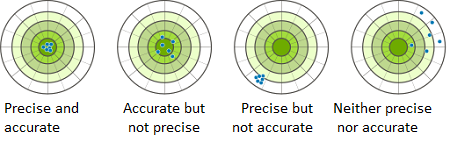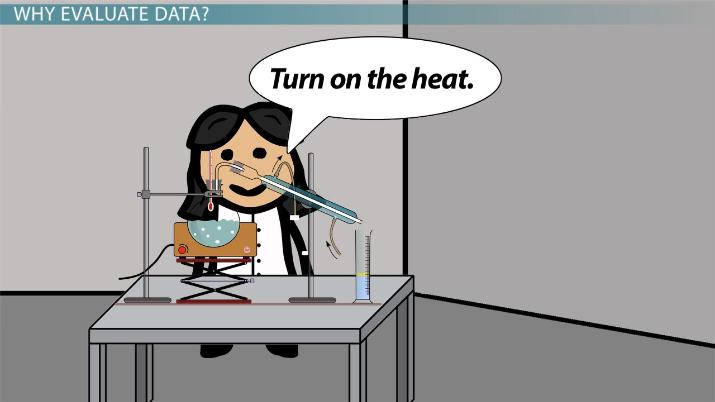# What is Accuracy and Precision in Data?

Christina Keathley, Elizabeth Friedl
• Author
Christina Keathley

Christina graduated with a Master's in biology from the University of Louisiana at Lafayette. She is a current PhD student in biology at Wake Forest University, and has been teaching undergraduate students biology for the last three years.

• Instructor
Elizabeth Friedl

Elizabeth, a Licensed Massage Therapist, has a Master's in Zoology from North Carolina State, one in GIS from Florida State University, and a Bachelor's in Biology from Eastern Michigan University. She has taught college level Physical Science and Biology.

This lesson will explain the meaning of accuracy and precision with respect to scientific measurements. Examples will be provided as well as a discussion of possible errors that can affect the accuracy and/or precision. Updated: 12/29/2021

Show

## Accuracy And Precision

To answer scientific questions, researchers collect samples from populations. A population represents an entire group of individuals. For instance, the human population includes all humans currently alive. A sample is a portion of that population, say the population of individuals from a single state.

How data is collected is important for the overall relevance of results. Michael is researching the average height of adult men. To measure every adult man on the planet would be impossible, so he takes a sample of 100 men from a local grocery store. Would the measurement he obtains represent the entire population? The answer depends on the precision and accuracy of his measurements. Precision is the ability to produce the same or similar results across multiple measurements. If all the men in Michael's sample were at or close to the same height, then his sampled measurements were precise. Accuracy is the distance a sampled mean's value is to the population's mean. If Michael found that his sample of men averaged at 6 feet in height and the average adult male height was 6 feet, then his results were accurate.

His measurements may also depend entirely on the equipment he's using to measure height. For instance, measuring height by asking individuals about their height versus using a ruler will produce different results. In this lesson, investigate accuracy and precision in more detail to answer the following questions:

• What is accuracy and what are examples of high and low accuracy?
• What is precision and what are examples of high and low precision?
• How might measurements produce accurate or precision errors?
• How might data precision and accuracy errors be reduced?### What is accuracy?

Accuracy is how far off a measurement is from its true value. A measured value that's far from a true value is inaccurate, while a measure that is close to a true value is accurate. High levels of accuracy are needed to correctly identify a population's value. A sample with an inaccurate mean will not correctly represent a trend seen within a population.

#### Measuring Accuracy

There are multiple ways to measure accuracy. One of the most utilized methods is to calculate a percent error. The percent error is a representation of how far off measured values are from the actual values and are represented by the following equation:

{eq}Error=\frac{x_{Actual}-x_{Measured}}{x_{Actual}} {/eq}

In this instance, a high percent error would mean that a value was far from the actual value and thus inaccurate. This equation assumes that the actual value is known or that a theoretical actual value is assumed.

Another method involves comparing the number of true values to false values. A true positive value is the number of individuals measured that accurately represent a condition within a population. A true negative value is the number of measured individuals within a population that does not have a condition and are predicted not to. False positive values occur when a condition is expected to exist but does not, and false negatives occur when a condition is expected to not occur but does.

Consider a study that is counting the number of black feral cats observed on a college campus. The following true and false values can thus be generated:

• True positive: A cat is predicted to be black, and it is black
• True negative: A cat is not predicted to be black and isn't
• False positive: A cat is predicted to be black but is not
• False negative: A cat is not predicted to be black but is

Previous literature states that the number of black cats in a colony is 10%. This study observed fifty cats and found that 15 were black and 35 were not. The prediction based on the previous data suggests that only 5 cats should be black, and thus the following values are found:

• True positives: 5
• True negatives: 35
• False positive: 0
• False negatives: 10

The level of accuracy can then be calculated using the following equation:

{eq}Accuracy = (\frac{TP+TN}{TP+FP+TN+FN})*100 {/eq}

Thus, the percent accuracy in this situation is 80% if the previous literature is true of the population.

### What is Precision?

Precision is the ability to produce the same or close to the same value multiple times. If someone was shooting at a target, their aim would be precise if all of their shots were at or near the exact same point. High precision coincides with low sample standard deviation. Standard deviation is a measurement of how widely spread a data set is. A sample standard deviation is specifically the spread of data of a particular sample and thus may not represent the true spread of data of the population.

#### Measuring Precision

Sample standard deviation is expressed by the following equation:

{eq}SD_{Sample}=\sqrt{\frac{\sum (x_{i}-\bar{x})^{2}}{n-1}} {/eq}

In this formula, {eq}x_{i} {/eq} represents an individual measurement while {eq}\bar{x} {/eq} is the mean of all measurements within a sample. The number of sampled individuals is represented by {eq}n {/eq}.

Consider the following example. Sarah wants to know which method of measuring distance is more precise. She takes a small piece of paper and measures it five times with an electric caliper and five times with a ruler. The values she obtains from her calipers are 4", 4.1", 3.8" 4" and 4.1" while with her ruler she measures 3.5", 4.2", 4.1", 4.4", and 3.8" respectively. The mean of her results, standard deviation, and overall precision is reported in the following table:

Tool Calipers Ruler
Mean 4 4
Standard Deviation 0.015 0.125
Degree of Precision High Low

Both methods returned the same mean which may lead one to believe that they are equally appropriate tools of measurement. However, the calipers returned similar values each time, returned a smaller deviation, and are thus more precise.

### Accuracy And Precision Examples

Investigating accuracy and precision examples is perhaps the best way to understand how these values differ. Below, investigate an example study to understand how data precision and accuracy compare.An error occurred trying to load this video.

Try refreshing the page, or contact customer support.

Coming up next: Using Appropriate Tools for Scientific Tests & Data Collection

### You're on a roll. Keep up the good work!

Replay
Your next lesson will play in 10 seconds
• 0:03 Why Evaluate Data?
• 1:12 Accuracy & Precision
• 3:30 Types of Errors
• 5:50 Lesson Summary
Save Save

Want to watch this again later?

Timeline
Autoplay
Autoplay
Speed Speed

To unlock this lesson you must be a Study.com Member.

#### What are some examples of accuracy?

Accuracy is the distance of a measured value to the true value. When throwing darts, hitting a bullseye is accurate while hitting an outer ring is not accurate.

#### What is accuracy and precision in measurement?

Accuracy refers to how close a set of measurements are to a goal. Precision is how close those measurements are to each other but not necessarily to the target measurement.

#### What is an example of low accuracy and high precision?

Low accuracy occurs when a value is far from the true value and high precision occurs when measured values are close together. If a scale was not calibrated it may show values that are higher than the actual weight of an object (and not accurate), but multiple measurements may return the same weight (and thus be precise).

### Register to view this lesson

Are you a student or a teacher?

Back

### Resources created by teachers for teachers

Over 30,000 video lessons & teaching resources‐all in one place.Video lessonsQuizzes & WorksheetsClassroom IntegrationLesson Plans

I would definitely recommend Study.com to my colleagues. It’s like a teacher waved a magic wand and did the work for me. I feel like it’s a lifeline.

Jennifer B.
TeacherCreate an account to start this course today
Used by over 30 million students worldwide